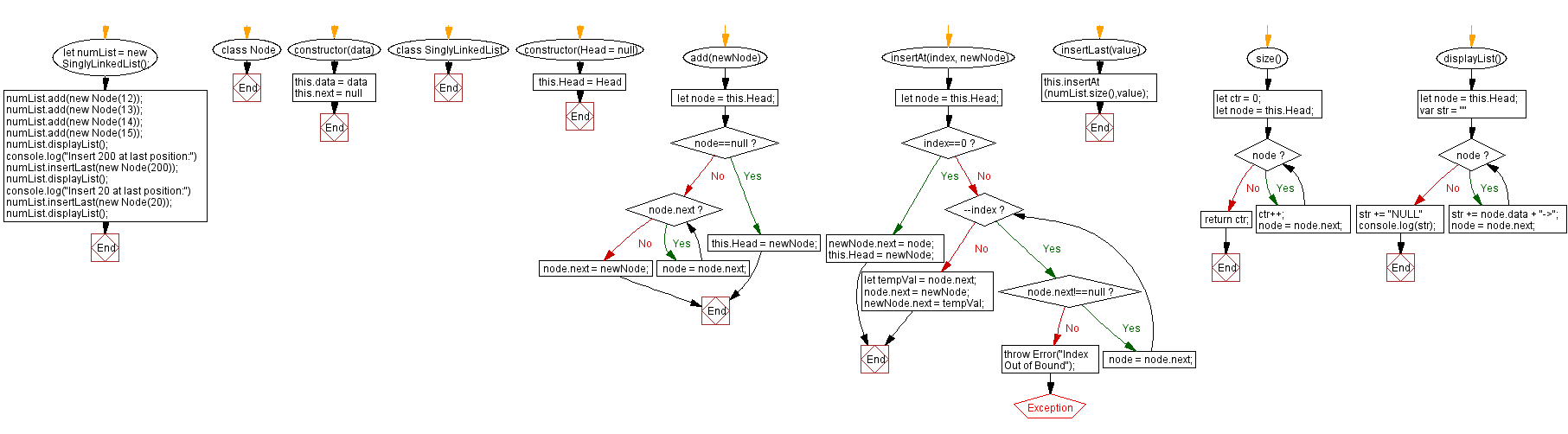# JavaScript Exercises: Insert a new node at the end of a Singly Linked List

## JavaScript Singly Linked List: Exercise-6 with Solution

Write a JavaScript program to insert a node at the end of a Singly Linked List.

Sample Solution:

JavaScript Code:

``````class Node {
constructor(data) {
this.data = data
this.next = null
}
}
}
if(node==null){
return;
}
while (node.next) {
node = node.next;
}
node.next = newNode;
}
insertAt(index, newNode){
if(index==0) {
newNode.next = node;
return;
}
while(--index){
if(node.next!==null)
node = node.next;
else
throw Error("Index Out of Bound");
}
let tempVal = node.next;
node.next = newNode;
newNode.next = tempVal;
}
insertLast(value) {
this.insertAt(numList.size(),value);
}
size() {
let ctr = 0;
while (node) {
ctr++;
node = node.next;
}
return ctr;
}

displayList(){
var str = ""
while (node) {
str += node.data + "->";
node = node.next;
}
str += "NULL"
console.log(str);
}
}
numList.displayList();
console.log("Insert 200 at last position:")
numList.insertLast(new Node(200));
numList.displayList();
console.log("Insert 20 at last position:")
numList.insertLast(new Node(20));
numList.displayList();

```
```

Sample Output:

```12->13->14->15->NULL
Insert 200 at last position:
12->13->14->15->200->NULL
Insert 20 at last position:
12->13->14->15->200->20->NULL
```

Flowchart:Live Demo:

See the Pen javascript-singly-linked-list-exercise-6 by w3resource (@w3resource) on CodePen.

Improve this sample solution and post your code through Disqus

Singly Linked List Previous: Insert a new node at the beginning of a Singly Linked List.
Singly Linked List Next: Get a node in an existing singly linked list.

What is the difficulty level of this exercise?

Test your Programming skills with w3resource's quiz.

﻿

## JavaScript: Tips of the Day

The unary operator

```let num = 10;

const increaseNumber = () => num++;
const increasePassedNumber = number => number++;

const num1 = increaseNumber();
const num2 = increasePassedNumber(num1);

console.log(num1);
console.log(num2);
```

The unary operator ++ first returns the value of the operand, then increments the value of the operand. The value of num1 is 10, since the increaseNumber function first returns the value of num, which is 10, and only increments the value of num afterwards.
num2 is 10, since we passed num1 to the increasePassedNumber. number is equal to 10(the value of num1. Again, the unary operator ++ first returns the value of the operand, then increments the value of the operand. The value of number is 10, so num2 is equal to 10.

Ref: https://bit.ly/323Y0P6

We are closing our Disqus commenting system for some maintenanace issues. You may write to us at reach[at]yahoo[dot]com or visit us at Facebook